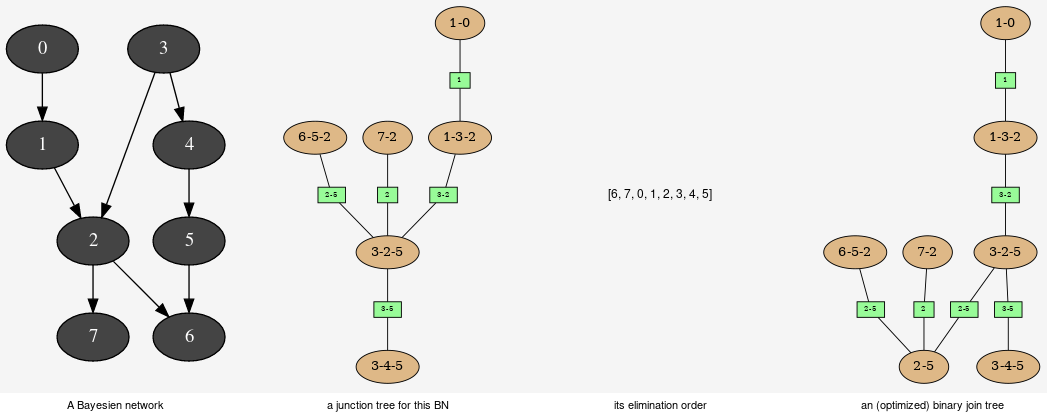# Explanation and analysis¶This tools aimed to provide some different views on the Bayesian network in order to explore its qualitative and/or quantitave behaviours.

class pyAgrum.JunctionTreeGenerator

JunctionTreeGenerator is use to generate junction tree or binary junction tree from bayesian networks.

Available ructors:
JunctionTreeGenerator() -> JunctionTreeGenerator
binaryJoinTree(JunctionTreeGenerator self, UndiGraph g, PyObject * partial_order=None)

binaryJoinTree(JunctionTreeGenerator self, DAG dag, PyObject * partial_order=None) -> CliqueGraph binaryJoinTree(JunctionTreeGenerator self, BayesNet bn, PyObject * partial_order=None) -> CliqueGraph

Computes the binary joint tree for its parameters. If the first parameter is a graph, the heurisitcs assume that all the node have the same domain size (2). If given, the heuristic takes into account the partial order for its elimination order.

Parameters: g (pyAgrum.UndiGraph) – a undirected graph dag (pyAgrum.DAG) – a dag bn (pyAgrum.BayesNet) – a BayesianNetwork partial_order (List[List[int]]) – a partial order among the nodeIDs the current binary joint tree pyAgrum.CliqueGraph
eliminationOrder(JunctionTreeGenerator self, UndiGraph g, PyObject * partial_order=None)

eliminationOrder(JunctionTreeGenerator self, DAG dag, PyObject * partial_order=None) -> PyObject eliminationOrder(JunctionTreeGenerator self, BayesNet bn, PyObject * partial_order=None) -> PyObject

Computes the elimination for its parameters. If the first parameter is a graph, the heurisitcs assume that all the node have the same domain size (2). If given, the heuristic takes into account the partial order for its elimination order.

Parameters: g (pyAgrum.UndiGraph) – a undirected graph dag (pyAgrum.DAG) – a dag bn (pyAgrum.BayesNet) – a BayesianNetwork partial_order (List[List[int]]) – a partial order among the nodeIDs the current elimination order. pyAgrum.CliqueGraph
junctionTree(JunctionTreeGenerator self, UndiGraph g, PyObject * partial_order=None)

junctionTree(JunctionTreeGenerator self, DAG dag, PyObject * partial_order=None) -> CliqueGraph junctionTree(JunctionTreeGenerator self, BayesNet bn, PyObject * partial_order=None) -> CliqueGraph

Computes the junction tree for its parameters. If the first parameter is a graph, the heurisitcs assume that all the node have the same domain size (2). If given, the heuristic takes into account the partial order for its elimination order.

Parameters: g (pyAgrum.UndiGraph) – a undirected graph dag (pyAgrum.DAG) – a dag bn (pyAgrum.BayesNet) – a BayesianNetwork partial_order (List[List[int]]) – a partial order among the nodeIDs the current junction tree. pyAgrum.CliqueGraph
class pyAgrum.EssentialGraph(*args)

Proxy of C++ pyAgrum.EssentialGraph class.

arcs(EssentialGraph self)
Returns: The lisf of arcs in the EssentialGraph list
children(EssentialGraph self, int id)
Parameters: id (int) – the id of the parent the set of all the children Set
edges(EssentialGraph self)
Returns: the list of the edges List
ids()

Deprecated method in pyAgrum>0.12.0. See nodes instead.

mixedGraph(EssentialGraph self)
Returns: the mixed graph pyAgrum.MixedGraph
neighbours(EssentialGraph self, int id)
Parameters: id (int) – the id of the checked node The set of edges adjacent to the given node Set
nodes(EssentialGraph self)
parents(EssentialGraph self, int id)
Parameters: id – The id of the child node the set of the parents ids. Set
size(EssentialGraph self)
Returns: the number of nodes in the graph int
sizeArcs(EssentialGraph self)
Returns: the number of arcs in the graph int
sizeEdges(EssentialGraph self)
Returns: the number of edges in the graph int
sizeNodes(EssentialGraph self)
Returns: the number of nodes in the graph int
skeleton(EssentialGraph self)
toDot(EssentialGraph self)
Returns: a friendly display of the graph in DOT format str
class pyAgrum.MarkovBlanket(*args)

Proxy of C++ pyAgrum.MarkovBlanket class.

arcs(MarkovBlanket self)
Returns: the list of the arcs List
children(MarkovBlanket self, int id)
Parameters: id (int) – the id of the parent the set of all the children Set
hasSameStructure(MarkovBlanket self, DAGmodel other)
Parameters: pyAgrum.DAGmodel – a direct acyclic model True if all the named node are the same and all the named arcs are the same bool
mb(MarkovBlanket self)
Returns: a copy of the directed graph pyAgrum.DiGraph
nodes(MarkovBlanket self)
Returns: the set of ids set
parents(MarkovBlanket self, int id)
Parameters: id – The id of the child node the set of the parents ids. Set
size(MarkovBlanket self)
Returns: the number of nodes in the graph int
sizeArcs(MarkovBlanket self)
Returns: the number of arcs in the graph int
sizeNodes(MarkovBlanket self)
Returns: the number of nodes in the graph int
toDot(MarkovBlanket self)
Returns: a friendly display of the graph in DOT format str